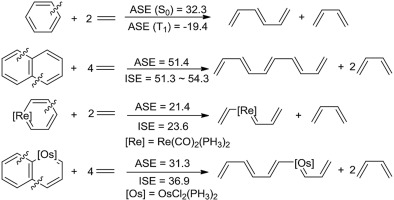# Direct energetic evaluation of aromaticity by cleaving the rings of cyclic compounds

Authors:
Ke An, Jun Zhu*
Journal:
J. Organomet. Chem.
Year:
2017
Volume:
864
FirstPage-LastPage:
81-87
TOC:Abstract:

Aromaticity, one of the central topics in chemistry, has attracted continuing interest of both experimentalists and theoreticians. Given that the original definition of aromaticity could be derived from the fact that the cyclic aromatic species are thermodynamically more stable than the corresponding acyclic conjugated ones, here we propose a direct evaluation of the aromatic stabilization energy (ASE) by following this definition to compute the energy difference between the cyclic compound and its corresponding acyclic one via a homodesmotic equation. Our results indicate that this method is able to produce a reasonable result in both the singlet ground state (S0) and the lowest triplet state (T1), evidenced by the correlations (R2 = 0.947 and 0.769 in the S0 and T1 states for organic cyclics and R2 = 0.851 in the S0 state for organometallics) between this method and the isomerization stabilization energy (ISE) method, and the magnetic indicator NICS(1)zz (R2 = 0.754 and 0.882 for organics in S0 and T1 states, respectively), thus providing an alternative to evaluate the aromaticity in the S0 and T1 states.

Doi:
10.1016/j.jorganchem.2018.01.038